Network Theorems - 1

# Network Theorems - 1 - Notes | Study Network Theory (Electric Circuits) - Electrical Engineering (EE)

 Table of contents1. Superposition Theorem2. Thevenin's Theorem3. Norton's Theorem4. Maximum Power Transfer TheoremFrequently Asked Questions (FAQs)1 Crore+ students have signed up on EduRev. Have you?

The fundamental theory on which many branches of electrical engineering, such as electric power, electric machines, control, electronics, computers, communications, and instrumentation are built is the Electric circuit theory. So here the network theorem helps us to solve any complex network for a given condition.

Note: All the theorems are only applicable to Linear Network only, according to the theory of Linear Network they follow the condition of Homogeneity & Additivity. So, before jumping to the theorems let's first understand the conditions for Linear Networks.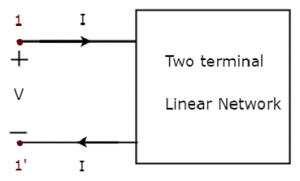An element is said to be linear if it satisfies homogeneity (scaling) property and additive (superposition) property.

1. Homogeneity Property

Let x be the input and y be the output of an element.

• x(t) → y(t)

If kx(t) is applied to the element, the output must be ky.

• kx(t) → ky(t)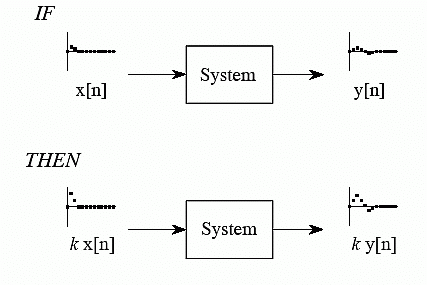Showing homogeneity property

x1(t) → y1(t),  x2(t) → y2(t)

If (x1(t) + x2(t)) is applied to the element, the output must be y1(t) + y2(t).

• x1(t) + x2(t) → y1(t) + y2(t)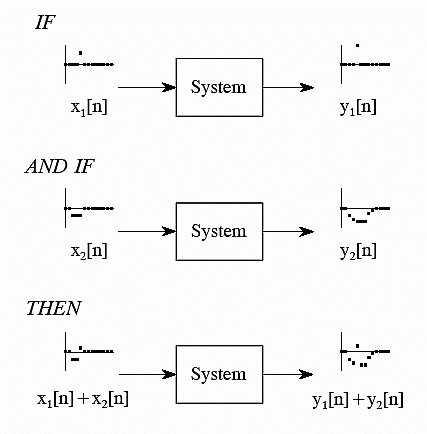If k(x1(t) + x2(t)) is applied to the element, the output must be k(y1(t) + y2(t)).

• kx1(t) + kx2(t) → ky1(t) + ky2(t)

So, for a network to qualify the application of various theorems must follow the conditions given above.

Let's study some amazing theorems:

1. Superposition Theorem

• It states that in a linear network with a number of independent sources, the response can be found by summing the responses to each independent source acting alone, with all other independent sources set to zero.
(a) Consider one independent source at a time while all other independent sources are turned off. It means that voltage source is replaced by short circuit and current source by open circuit.
(b) Leave dependent sources in the circuit as they are controlled by other variables.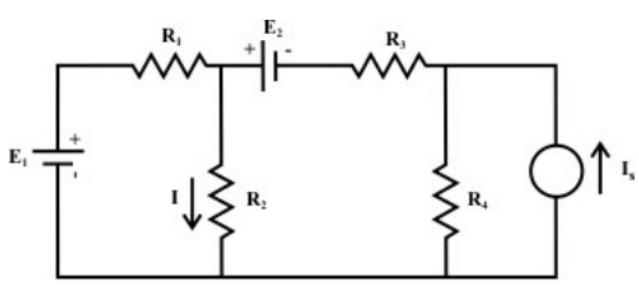Procedure for using the superposition theorem

• Step-1: Retain one source at a time in the circuit and replace all other sources with their internal resistances.
• Step-2: Determine the output (current or voltage) due to the single source acting alone.
• Step-3: Repeat steps 1 and 2 for each of the other independent sources.
• Step-4: Find the total contribution by adding algebraically all the contributions due to the independent sources.

So for above given circuit the total response or say current I through register R2 will be equal to the sum of individual response obtained by each source.

I = I'|due to E1(alone) + I''|due to E2(alone) + I'''|due to Ix(alone)

Question for Network Theorems - 1
Try yourself: In superposition theorem, when we consider the effect of one voltage source, all the other voltage sources are ____________.

Removing of Active Element in Superposition Theorem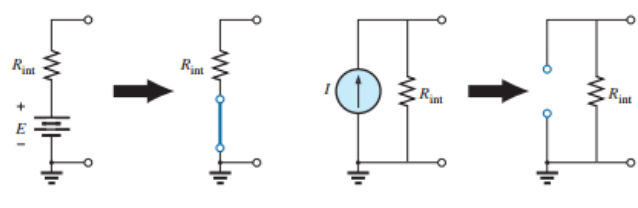• Limitation Superposition cannot be applied to power effects because the power is related to the square of the voltage across a resistor or the current through a resistor.
• The squared term results in a nonlinear (a curve, not a straight line) relationship between the power and the determining current or voltage.

Example: Here in the following electrical circuit, we will find the current flowing through the 10 Ω resistor using the superposition theorem.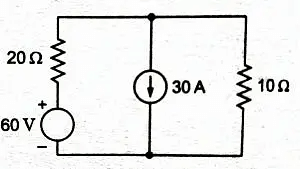Here at first let’s consider the 30 A current source. So we will leave the 30 A current source as it is in the circuit and replace the 60 V voltage source with the short circuit as shown below.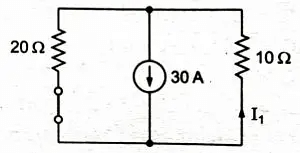Now the current through 10 Ω resistor is calculated as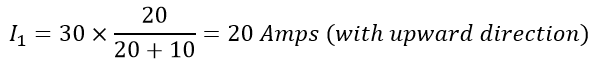[The I1 is calculated using the current divider.]

Now let’s consider a 60 V voltage source. So we will leave the 60 V voltage source as it is and replace the 30 A current source with the open circuits shown below.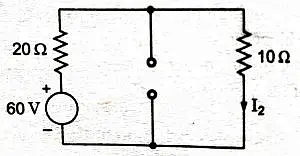Then current through 10 Ω resistor is calculated as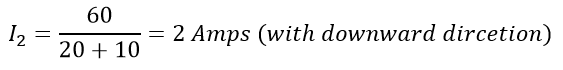Finally, the total current flowing through the 10 Ω resistor is the algebraic sum of I1 and I2.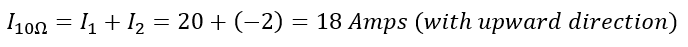2. Thevenin's Theorem

Thevenin’s theorem states that any two output terminals of an active linear network containing independent sources (it includes voltage and current sources) can be replaced by a simple voltage source of magnitude VTH in series with a single resistor RTH where RTH is the equivalent resistance of the network when looking from the output terminals A & B with all sources (voltage and current) removed and replaced by their internal resistances and the magnitude of VTH is equal to the open circuit voltage across the A & B terminals.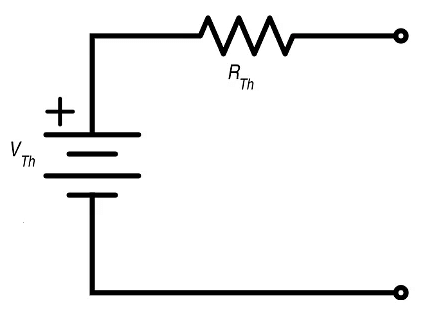Thevenin Circuit

The procedure for applying Thevenin’s theorem

• Calculate / measure the open circuit voltage. This is the Thevenin Voltage (VTH).
• Open current sources and short voltage sources.
• Calculate /measure the Open Circuit Resistance. This is the Thevenin Resistance (RTH).
• Now, redraw the circuit with measured open circuit Voltage (VTHin Step (2) as voltage source and measured open circuit resistance (RTH) in step (4) as a series resistance and connect the load resistor which we had removed in Step (1). This is the equivalent Thevenin circuit of that linear electric network or complex circuit which had to be simplified and analyzed by Thevenin’s Theorem.
• Now find the Total current flowing through the load resistor by using the Ohm’s Law: IT = VTH / (RTH + RL).

Example: Find current flowing through 1 \OmegaΩ resistor.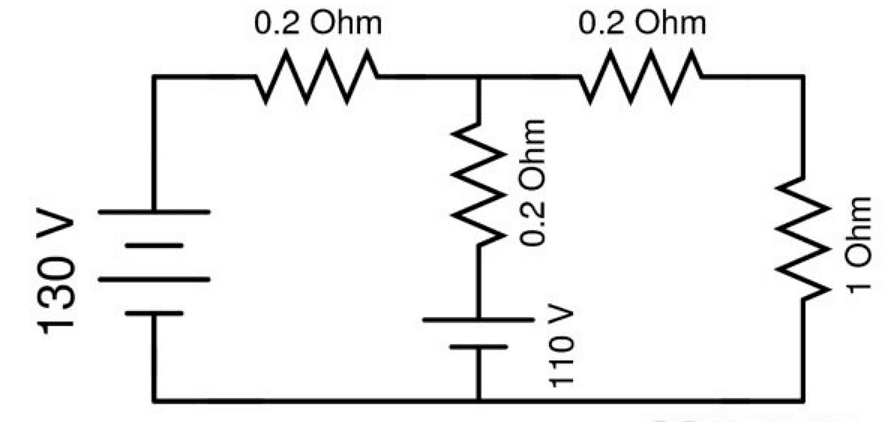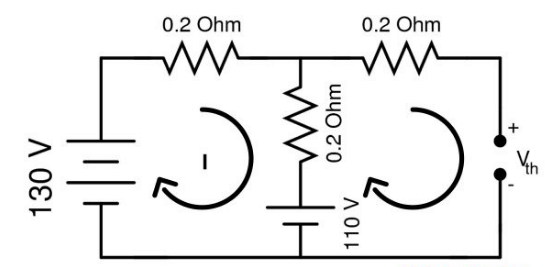Open Load Resistor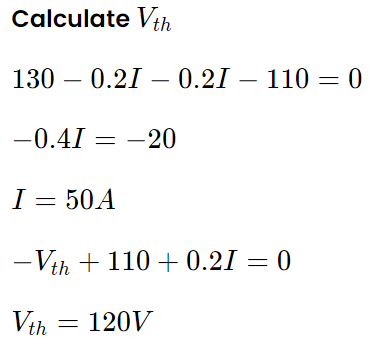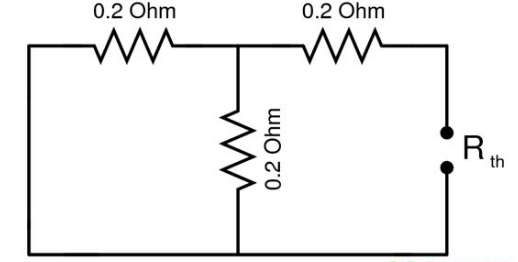Voltage Source are shorted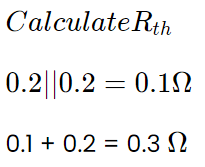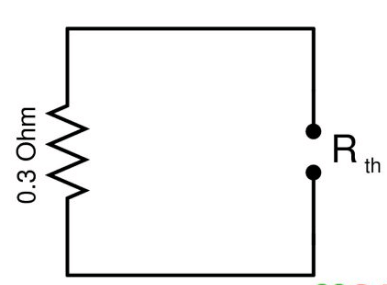Equivalent Circuit to find Rth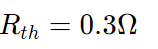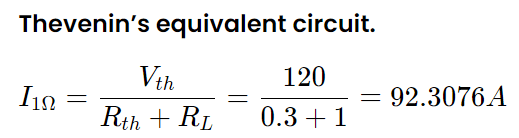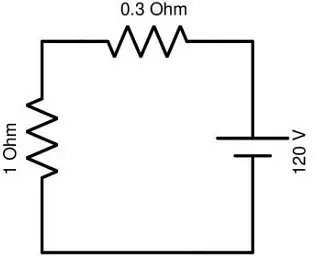Question for Network Theorems - 1
Try yourself: Find the value of Vth from the below circuit.Question for Network Theorems - 1
Try yourself:Calculate the Thevenin resistance across the terminal AB for the following circuit.Question for Network Theorems - 1
Try yourself:Calculate Vth for the given circuit.3. Norton's Theorem

• Norton’s theorem states that any linear network containing can be replaced by a current source and a parallel resistor.
• RN = RTH is the equivalent resistance of the network when looking from the output terminals A & B with all sources (voltage and current) removed and replaced by their internal resistances and the magnitude of VTH is equal to the open circuit voltage across the A & B terminals.
• IN is the Load current.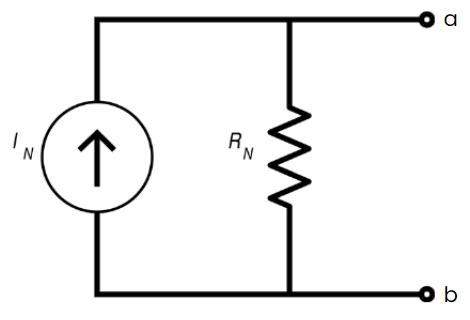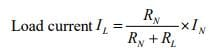Example: For the given circuit, determine the current flowing through 10 Ω resistor using Norton’s theorem.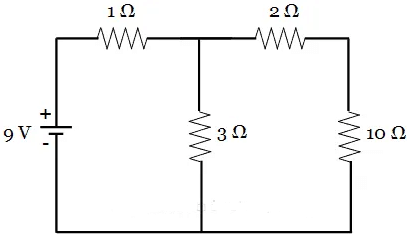Since the question here, is to determine the current through 10 Ω resistor, it is considered as the load.

(a) To find Norton’s current, Remove the load resistor(10 Ω), short it with a wire and the circuit is redrawn as below.

In this circuit, we need to find the current IN, which is Norton’s current flowing from a to b. To find the value of IN, it is necessary to determine the total current in the circuit.

If you observe the circuit, 3 Ω resistor and 2 Ω resistor are in parallel with each other. This parallel combination is in series with 1 Ω resistor. Thus,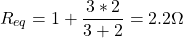Now, the total current IT is given by,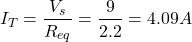The current through the 2 Ω resistor (or Norton’s current IN) is obtained by applying current division rule: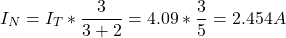(b) To find Norton’s resistance, remove the load resistor, short the voltage source and circuit is redrawn as below.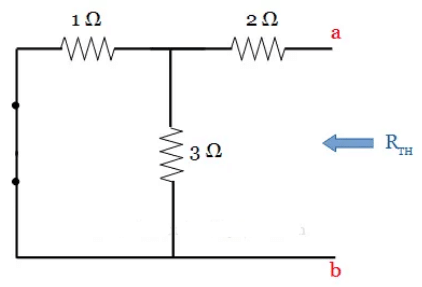In this circuit, we can observe that the 2 Ω resistor is in series with the parallel combination of 1 Ω and 3 Ω resistors. Thus the equivalent value of resistance is obtained as,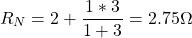(c) Norton’s Equivalent Circuit. It is drawn by connecting Norton’s voltage IN, Norton’s resistance RN and load resistor in series, as shown below: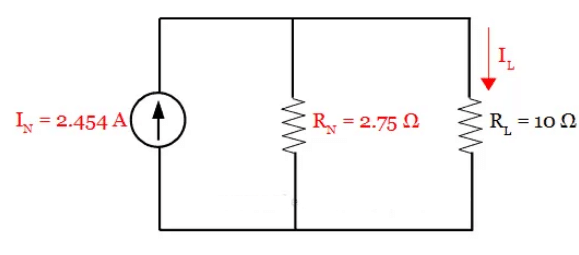From this circuit, the current through the load RL = 10 Ω resistor is obtained using current division rule. It is given by,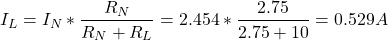4. Maximum Power Transfer Theorem

The maximum power transfer theorem states that, to obtain maximum external power from a source with a finite Internal Impedance (Say Resistance) the resistance of the load must equal to the resistance of the source as viewed from its output terminals.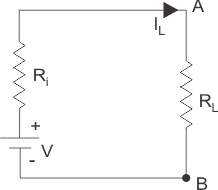Power delivered to the load resistance: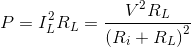To find the maximum power, differentiate the above expression with respect to resistance RL and equate it to zero. Thus,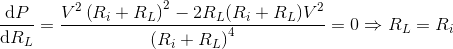Thus in this case, the maximum power will be transferred to the load when load resistance is just equal to internal resistance of the battery.

Results of Maximum Power Transfer:

• The maximum power transfer takes place when the load resistance R= Rth
• The maximum power transferred to the load Pmax = PL (R= Rth) = (Vth)2/ 4Rth
• The efficiency of power transfer η = PL/Ps
• where PL- Power delivered to the Load
• Ps- Power Generated by Source

Note: Maximum power transfer condition results in 50 percent efficiency in Thevenin equivalent, however much lower efficiency in the original circuit.

Example: The maximum power drawn by the load RL in the below circuit will be: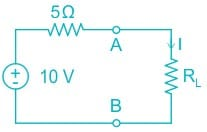Calculation:

Here Rth= 5 ohm and Vth= 10 V and RL= 5 ohm.

So Thevenin equivalent would be: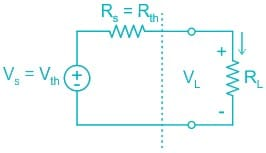So, power across load can be calculated by calculating current I across RL.

I = Vth/Req

Req = 5 + 5

= 10 ohm

I = 10/10

I = 1 A.

So, power across RL = I­2RL

= 1 × 5

= 5 W

Q.1. What is the superposition theorem?

Superposition theorem is a circuit analysis theorem that is used to solve the network where two or more sources are present and connected.

Q.2. Is the superposition theorem valid for AC circuits?

The superposition theorem is valid for AC circuits.

Q.3. Is the superposition theorem applicable to power?

The requisite of linearity indicates that the superposition theorem is only applicable to determine voltage and current, but not power. Power dissipation is a nonlinear function that does not algebraically add to an accurate total when only one source is considered at a time.

Q.4. Can the superposition theorem be applied to non-linear circuits?

No, the superposition theorem can only be applied to non-linear circuits.

Q.5. Why do we use the superposition theorem?

The superposition theorem is very important in circuit analysis because it converts a complex circuit into a Norton or Thevenin equivalent circuit.

The document Network Theorems - 1 - Notes | Study Network Theory (Electric Circuits) - Electrical Engineering (EE) is a part of the Electrical Engineering (EE) Course Network Theory (Electric Circuits).
All you need of Electrical Engineering (EE) at this link: Electrical Engineering (EE)

## Network Theory (Electric Circuits)

23 videos|63 docs|60 tests

## Network Theory (Electric Circuits)

23 videos|63 docs|60 tests

Track your progress, build streaks, highlight & save important lessons and more!

,

,

,

,

,

,

,

,

,

,

,

,

,

,

,

,

,

,

,

,

,

;